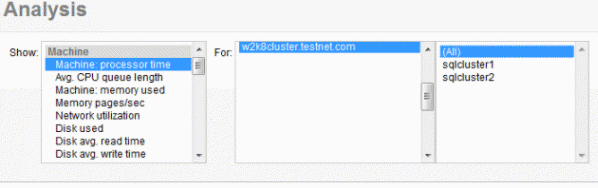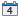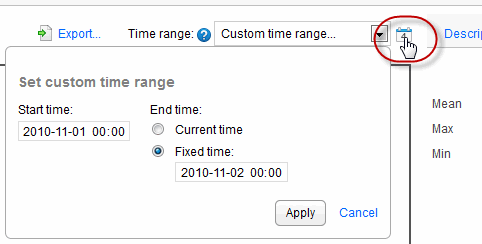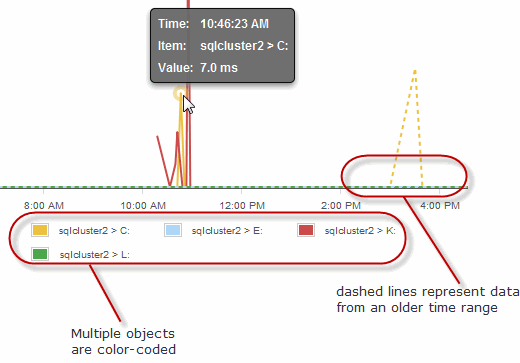# Working with metrics in SQL Monitor 3.2 and earlier

## To view an analysis graph

1. Choose the performance metric you want to plot from the Show drop-down list. Metrics are grouped into Machine, SQL Server, Database and Custom metric categories.
2. Select the object to plot from the For list.
For some types of metric, you can plot all the objects together. The example below shows Machine: processor time for all machines in the clusters on the selected server.3. Choose a time range. By default, the graph shows the last 10 minutes, but you can select a different fixed value from the Time range drop-down list.
4. To specify a particular time range, click the Set custom time range iconand enter the start and end time.## Viewing values on the graph

Each graph line represents the performance of a selected object. SQL Monitor plots up to a maximum of 500 data points on each graph and draws straight lines between consecutive points. For short time ranges (less than 10 minutes) the graph displays fewer data points. For longer time ranges, more data points are displayed, so that these graphs can still provide a detailed overview of changes in values. Move your mouse pointer over a line on the graph to view a tooltip showing the time, object and value at that data point.

If you are comparing data for different time ranges, the graph line for the older time range is displayed as a dashed line. If you are comparing data for multiple objects, a color-coded key is displayed below the graph.## Plotting data points from collected values or calculated values

Data points are plotted differently depending on whether values are collected or calculated:

• collected values - data points represent the actual collected values. For example, Disk used simply displays the raw values provided by PerfMon. To optimize performance, some data points are not plotted if a long time range is selected.
• calculated values - SQL Monitor has to carry out its own calculation on raw data to generate a value. For example, to work out Transactions/sec, the number of transactions between data points is divided by the number of seconds between data points. This averaging has the effect of smoothing out fluctuations.

Didn't find what you were looking for?